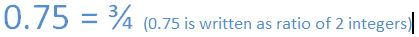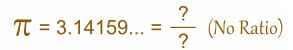# JavaScript > Math Reference

In this section we shall learn Math Object Properties & Math Object Methods in JavaScript.

Math Object:

By using Math object we can perform mathematical tasks, Math object is not a constructor and the properties of Math can be called by using Math as an object.

Rational Number:

A Rational number is a real number which can be written as a simple fraction (A ratio of 2 integers).

EXAMPLE:Irrational Number:

An Irrational number is a real number which cannot be written as simple fraction  (A ratio of 2 integers).

EXAMPLE:Transcendental Number:

A Transcendental number is a real or complex number that is not algebraic. The best known transcendental numbers are π and e. Where the value of π (Pie) is approximately 3.14145 and e (Euler's Number)is 2.7182.

Complex Number:

A complex number is a number that can be expressed in the form of x+yi, where x and y are the real numbers and iis the imaginary unit, which satisfies the equation i2=-1. In the expression x+yi, x is the real part and y is the imaginary part of the complex number.

Logarithm:

The logarithm is the inverse operation to exponentation, that means the logarithm of a number is the exponent to another fixed value, the base must be raised to produce the number. In very simple cases the logarithm counts repeated multiplication. The logarithm can be calculated for any two positive real numbers b and x, where b is not equal to 1.

7 posts found